Get instant live expert help with Excel or Google Sheets“My Excelchat expert helped me in less than 20 minutes, saving me what would have been 5 hours of work!”

#### Post your problem and you’ll get Expert help in seconds.

Your message must be at least 40 characters
Our professional Expert are available now. Your privacy is guaranteed.

# Using COUNTIF to Find a Running Count of an Occurrence in a List

We can do a running (continuous) count of an occurrence in a list by using the COUNTIF function in combination with the IF function. The steps below will walk you through the procedure.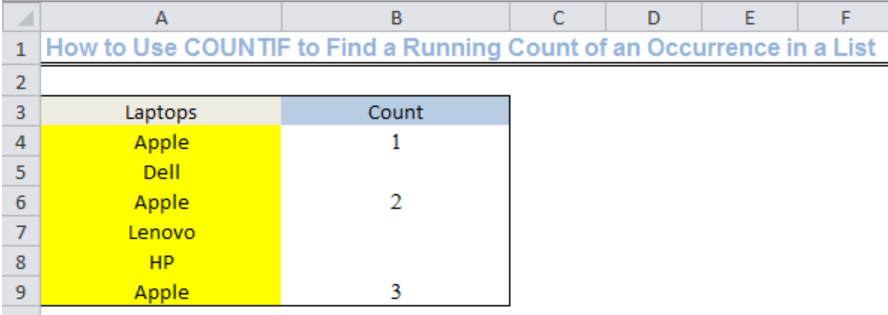Figure 1: Using COUNTIF to Find the Running Count of an Occurrence in the List

## Setting up the Data

• We will name Cell A3 as Laptops
• We will input the names of laptops into Column A from Cell A4 to Cell A9
• We will use Column B for the count and name Cell B3 as count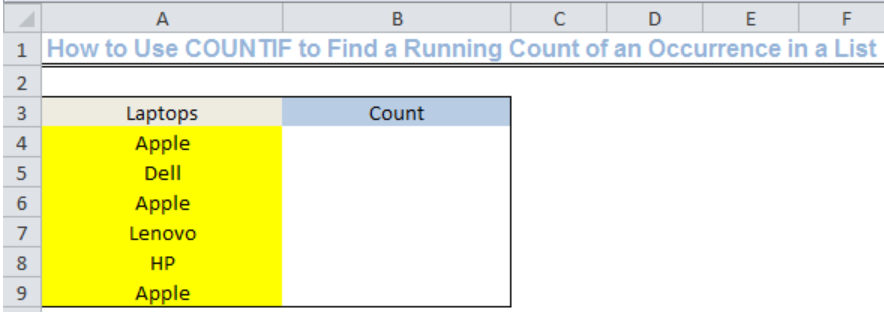Figure 2: Setting up the Data

## General Formula

• `=IF(cell containing the item="item",COUNTIF(\$A\$4:A4,"item"),"")`

## Formula

• `=IF(A4="Apple",COUNTIF(\$A\$4:A4,"Apple"),"")`

## Using COUNTIF to Find the Running Count of an Occurrence in a List

• We will insert the formula above into Cell B4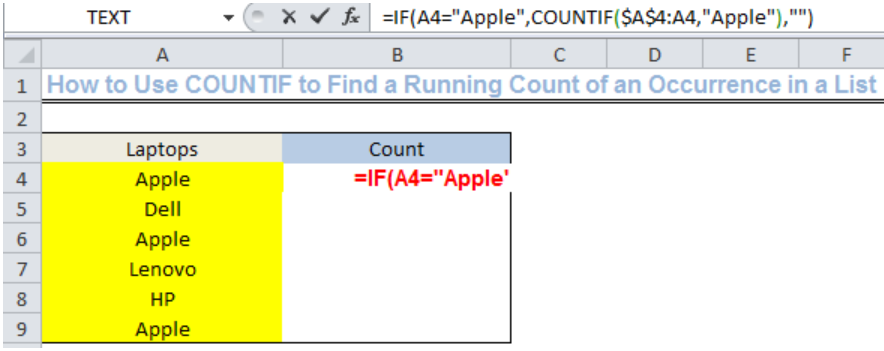Figure 3: Using COUNTIF to Find the Running Count of an Occurrence in a List

• Now, we will press ENTER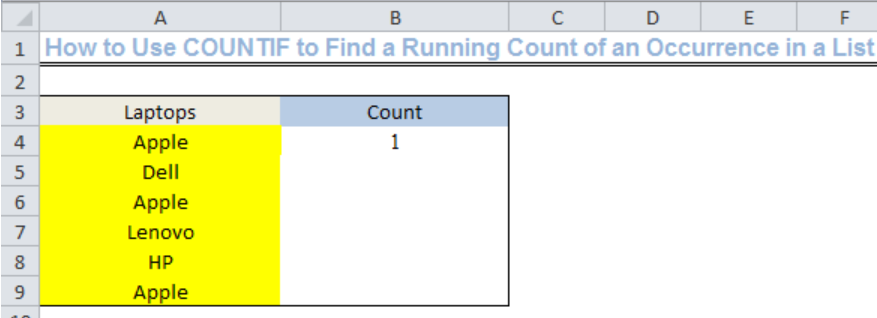Figure 4: Result of COUNTIF in Finding the First Count of an Occurrence in a List

• Now, we will use the drop-down feature to get the continuous count of APPLE in the list from Cell B4.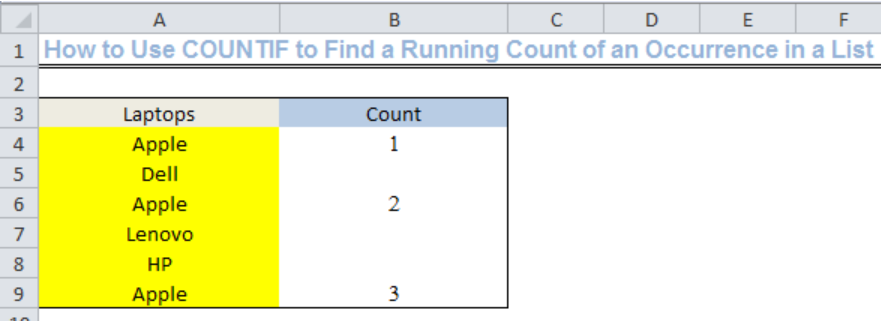Figure 5: Using COUNTIF to Find the Running Count of Apple in the List

## Explanation

We can create a running count of an occurrence in a list by using the COUNTIF function with a mixed reference to get a running or continuous total for an item. The mixed reference is present within the COUNTIF function; COUNTIF(\$A\$4:A4,”Apple”). The dollar sign locks the left side of the range (\$A\$4:A4) and leaves the right (A4) relative. This creates an expanding range so that once the formula is copied, the first cell in the reference will be locked and the second reference is able to expand to accommodate each new row. The COUNTIF then counts the number of cells in the range that are equal to APPLE with the result being the running count.

Whenever COUNTIF fails to generate a count, IF returns an empty string (“”).

## Instant Connection to an Expert through our Excelchat Service

Most of the time, the problem you will need to solve will be more complex than a simple application of a formula or function. If you want to save hours of research and frustration, try our live Excelchat service! Our Excel Experts are available 24/7 to answer any Excel question you may have. We guarantee a connection within 30 seconds and a customized solution within 20 minutes.

### Did this post not answer your question? Get a solution from connecting with the expert.Another blog reader asked this question today on Excelchat:
Solution examplesHello, I am trying to create a total color column? Can anyone help?
Solved by I. A. in 60 minsI need a formula to check text values in a range and output one of three possible outputs into a cell. ie: If all text values in cell range = A, then Output 1. If all text values in cell range = B, then Output 2. If there is a mix of A and B values in cell range, then Output 3.
Solved by M. J. in 60 minsI need a formula to count the number of rows that contain at least one of two possible phrases, but not give me the total number of instances that both phrases occur overall.
Solved by E. B. in 33 minsHello, I have a spreadsheet with 3159 rows. One column consists of 9-digit numbers in each of the 3159 rows. I need the formula that can calculate how many of those numbers appear more than once.
Solved by D. H. in 56 minshello i need help creating a formula, if a value does not exsits put 0, if a value exist do nothing
Solved by M. F. in 9 mins## Subscribe to Excelchat.coAnother blog reader asked this question today on Excelchat: